• 符号计算
千次阅读
2022-02-08 18:13:44

作为强大的数学软件，matlab有着许多方便快捷的符号运算，计算一元二次方程的根、合并同类项、符号表达式化简、求导、求极限、求积分等等，这些符号运算在matlab中只需调用函数。

符号运算的用法总结如下:

1、建立符号对象：sym 和 syms

符号对象: 在进行符号运算时，必须先定义基本的符号对象，可以是符号常量、符号变量、符号表达式等。

符号表达式: 含有符号对象的表达式。

符号矩阵/数组: 元素为符号表达式的矩阵/数组。

2、建立符号表达式：

(1) 用 sym 函数直接建立符号表达式。
例：>> y=sym('sin(x)+cos(x)') %老版
>> y=str2sym('sin(x)+cos(x)') %新版（2019）
(2) 使用已经定义的符号变量组成符号表达式。
>>  syms x;
>>  y=sin(x)+cos(x)

3、计算行列式：det(A)

例：>> A=sym('[x,y,x+y;y,x+y,x;x+y,x,y]'), det(a)

4、查找符号变量：findsym(expr,N)

5、替换符号表达式中的量：subs(f,x,a）

6、因式分解：factor(f)，factor(N)

7、函数展开：expand(f)

8、合并同类项：collect(f)，collect(f,y)

9、函数简化：symplify(f)

10、通分约分：[N,D]=numden(f)

11、多项式提取x变成嵌套形式：horner(f)

12、计算极限：limit(f,x,a,'right/left')

13、计算导数：diff(f,y,n)

14、计算积分：int(f,y,a,b)

15、符号求和：symsum(f,y,a,b)

16、求反函数：finverse(f,t)matlab
更多相关内容
• MATLAB编程符号计算 行命令a=sym('pi','d')，则对于变量a的描述 分别使用 sym 和syms创建符号表达式“sin(x)+cos(y)”
• 文件是MATLAB学习过程中自己总结的符号计算部分思维导图，方便复习使用，有png和mmax版，方便用思维导图软件编辑
• 利用matlab求解下列各题 计算下列极限 1 2 3 2求下列函数的导数 1求的一阶导数 2设求 3已知求y' 3求下列积分 1 2 4解微分方程 5求的6阶Taylor展开式 6求方程在1附近的近似根 7设 求1, (2, (3)A的特征值
• 第七讲 符号运算 1符号计算基础 2微积分 3简化方程表达式 4符号积分变换 5解方程 6图示化符号函数计算器 其他函数 符号表达式的因式分解与展开的函数调用格式为 factor(s)对符号表达式s分解因式 expand(s)对符号...
• 实验三 MATLAB的符号运算 一 实验目的 1.掌握符号对象的创建及符号表达式化简的基本方法 2.掌握符号微积分符号方程的求解的基本方法 二 实验装置 计算机 三 实验内容 1.符号对象的创建 (1) 建立符号变量 使用sym函数...
• 1.基本符号运算2.将变量定义为符号或者，在一行中定义多个符号变量3.查找符号变量4.字符串处理实用程序5.检查变量名是否可以接受6.变精度算法7.转换功能8. 方程的解9.简单的图形功能10.积分和求和变换等等.............matlab
• 符号运算是 MATLAB数值计算的扩展，在运算过程中以符号表达式或符号矩阵为运算对象，实现了符号计算和数值计算的相互结合，使应用更灵活。 符号表达式与数值表达式 符号表达式与数值表达式的相互转换主要是通过...

在数学、物理学及力学等各种学科和工程应用中，经常还会遇到符号运算的问题。在MATLAB中，符号运算是为了得到更高精度的数值解，但数值的运算更容易让读者理解，因此在特定的情况下，分别使用符号或数值表达式进行不同的运算。

符号运算是 MATLAB数值计算的扩展，在运算过程中以符号表达式或符号矩阵为运算对象，实现了符号计算和数值计算的相互结合，使应用更灵活。

## 符号表达式与数值表达式

符号表达式与数值表达式的相互转换主要是通过函数evalsym实现的。其中，eval函数用于将符号表达式转换成数值表达式，而函数sym用于将数值表达式转换成符号表达式。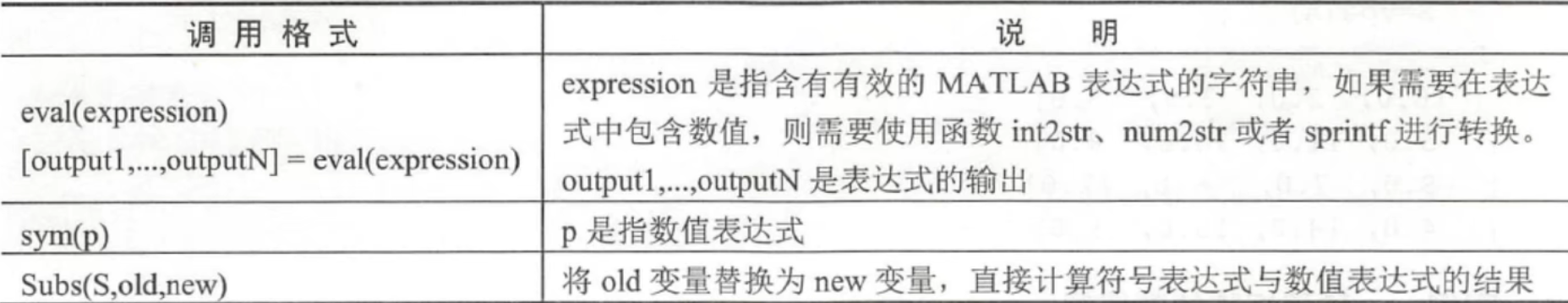符号表达式与数值表达式分别使用函数digits和函数vpa来进行精度设置。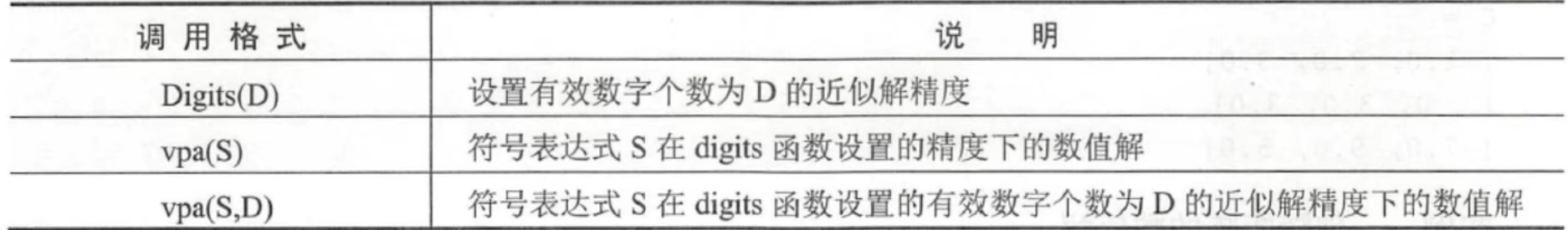## 符号矩阵

符号矩阵和符号向量中的元素都是符号表达式，符号表达式是由符号变量与数值组成的。

符号矩阵中的元素是任何不带等号的符号表达式,各符号表达式的长度可以不同。符号矩阵中以空格或逗号分隔的元素指定的是不同列的元素而以分号分隔的元素指定的是不同行的元素。

### 符号矩阵的生成

1.直接生成法

直接输入符号矩阵时，符号矩阵的每一行都要用方括号括起来，而且要保证同一列的各行元素字符串的长度相同，因此在较短的字符串中要插入空格来补齐长度，否则程序将会报错。

2.用sym函数创建符号矩阵

### 符号矩阵的运算

在 MATLAB中，数值矩阵不能直接参与符号运算，所以必须先转化为符号矩阵。

与数值矩阵一样，符号矩阵可以进行转置、求逆等运算，但符号矩阵的函数与数值矩阵的函数不同。

(1)符号矩阵的转置运算

符号矩阵的转置运算可以通过符号“ ' ”或函数transpos来实现，其调用格式如下。

B=A.'

B=transpos(A)

(2)符号矩阵的行列式运算

符号矩阵的行列式运算可以通过函数determ或det来实现，其中矩阵必须使用方阵，调用格式如下。

d=det(A)

(3)符号矩阵的逆运算

符号矩阵的逆运算可以通过函数inv来实现，其中矩阵必须使用方阵，调用格式如下。

inv(A)

(4)符号矩阵的求秩运算

符号矩阵的求秩运算可以通过函数rank来实现,调用格式如下。

rank(A)

(5)符号矩阵的常用函数运算

符号矩阵的特征值、特征向量运算：可以通过函数eigeigensys来实现。

符号矩阵的奇异值运算：可以通过函数svdsingavals来实现。

符号矩阵的若尔当(Jordan)标准形运算：可以通过函数jordan来实现。。。。

符号工具箱中还提供了符号矩阵因式分解、展开、合并、简化及通分等符号操作函数。

(1)因式分解

符号矩阵因式分解通过函数factor来实现，其调用格式如下。

factor(S)

(2)符号矩阵展开

符号多项式的展开可以迪过函数expand来实现，其调用格式如下。

expand(S)

对符号矩阵的各元素的符号表达式进行展开。此函数经常用在多项式的表达式中，也常用在三角函数、指数函数、对数函数的展开中。

(3)符号简化

符号简化可以通过函数simple和 simplify来实现，其调用格式如表所示：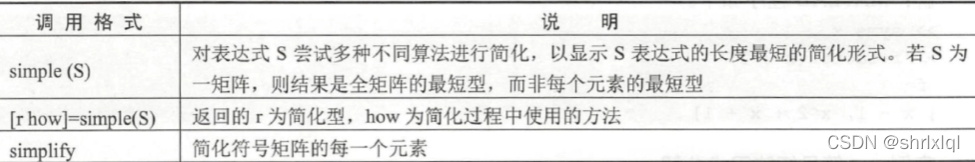(4)分式通分

求解符号表达式的分子和分母可以通过函数numden来实现，其调用格式如下。

【n,d】=numden(A)

把A的各元素转换为分子和分母都是整系数的最佳多项式型。

(5)符号表达式的“秦九韶型”重写

符号表达式的“秦九韶型”重写可以通过函数 horner(P)来实现，其调用格式如下。

horner(P）

将符号多项式转换成嵌套形式表达式。

展开全文matlab
• ## Python 符号运算

千次阅读 2020-12-12 14:57:53
背景介绍： Sugar 起初在 MultiMCU EDU 上写的都是有飞控、嵌入式操作系统、控制算法类的推文。 最近推的 Matlab 和 Python 似乎与 MCU...本篇 Sugar 就来说一说 Python 中的符号运算。 sympy 一、安装 sympy 模块 Su.

背景介绍：
Sugar 起初在 MultiMCU EDU 上写的都是有飞控、嵌入式操作系统、控制算法类的推文。
最近推的 Matlab 和 Python 似乎与 MCU 不在一个世界里，其实不然。机器人身上的 MCU 所跑的程序是有运行控制算法的，而控制算法的学习必须有扎实的数学功底。
当代学数学除了 Matlab 工具可用，还有开源的 Python 模块。学数学必须借助好工具，把抽象变具象才能理解。本篇 Sugar 就来说一说 Python 中的符号运算。

## sympy

一、安装 sympy 模块

Sugar 使用 Python 3.8.6 安装 sympy 模块，安装命令如下：

pip install sympy


二、sympy 使用

1、symbols() 函数定义符号

import sympy as sy
x,y = sy.symbols("x y")


如上定义 xy 为符号变量，可以用 type() 函数查看变量类型，如下：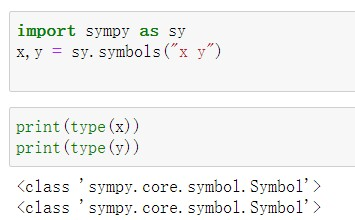2、字符表达式输出方法：

f"...{}"字符串前加 f{} 里的内容格式化输出。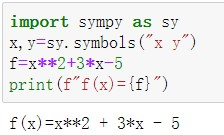3、符号表达式的数值解

subs() 函数用于将表达式里的变量替换成数值。
f字符串里凡是要求值的地方都用 {} 括起来。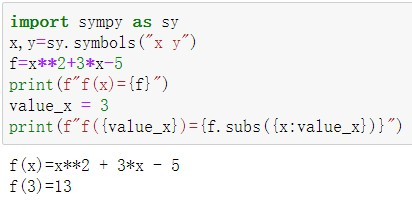4、变量替换（适用于复合函数）

subs() 函数可以将表达式里的变量替换成其他符号表达式。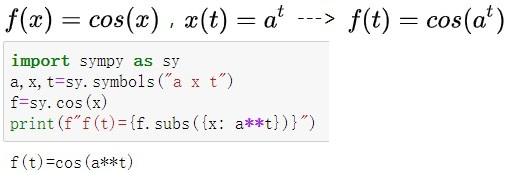5、算术表达式求值

Rational(a,b) 表示分数 a/b
sqrt(a) 表示求 a 的平方根。
evalf() 函数用于求算数表达式的数值解。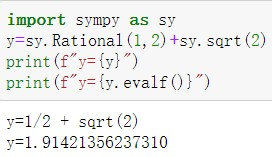6、输出更易懂的表达式

init_printing()pprint() 就能以更易懂的方式输出符号表达式。7、输出更专业的表达式

这里要用到 jupyter notebook，关于 jupyter 的安装和使用 Sugar 下面会单独说。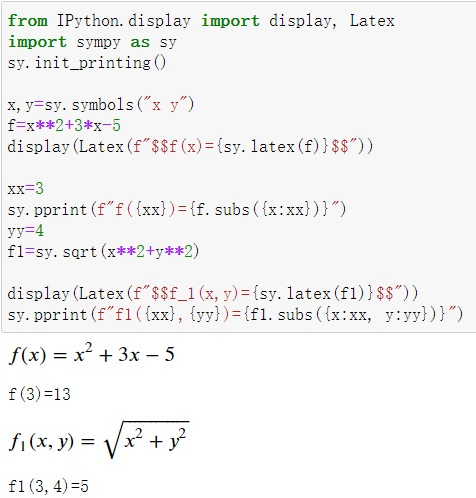sympy 模块更多强大的功能可以参考简书上的《Python科学计算利器——SymPy库》，以上内容 Sugar 有参照知乎上的《sympy: 符号运算-1》，在知乎专栏《sympy:符号运算》中有更多内容。本篇意在让初学者简单入门，选得都是简单好用的示例，避免初学者看一大篇长文引起畏难情绪。

## jupyter

jupyter notebook 是用来学代码的不二之选，相当于一个活的可运行的代码笔记，功能上类似于 Matlab 的实时脚本。

一、安装 jupyter

pip 安装命令 pip install jupyter

二、简单配置和使用

1、换 jupyter notebook 启动目录
(1) 在 D 盘新建文件夹 D:\jupyter_notebook
(2) 生成配置文件，命令是：jupyter notebook --generate-config
(3) 修改配置文件（搜索关键词 c.NotebookApp.notebook_dir），如下图：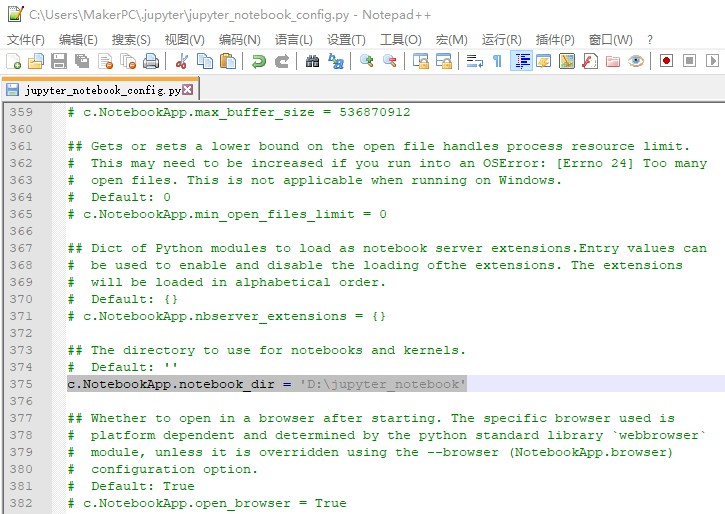2、基本使用：在 jupyter 里用 python3 输出公式。
(1) 在 Windows PowerShell 里输入命令 jupyter notebook 打开 jupyter 网页，然后新建 Python3 文件，如下图：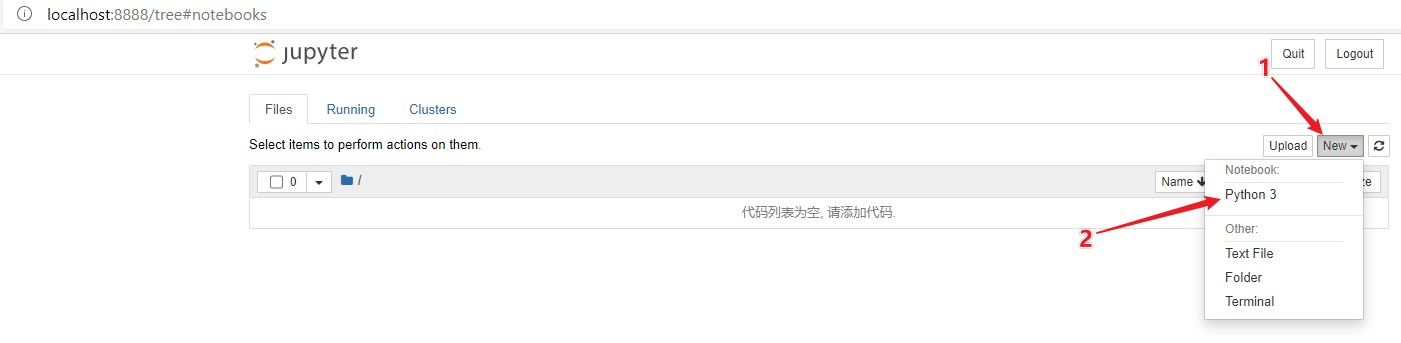(2) 自动跳转到 Python3 页面，如下图：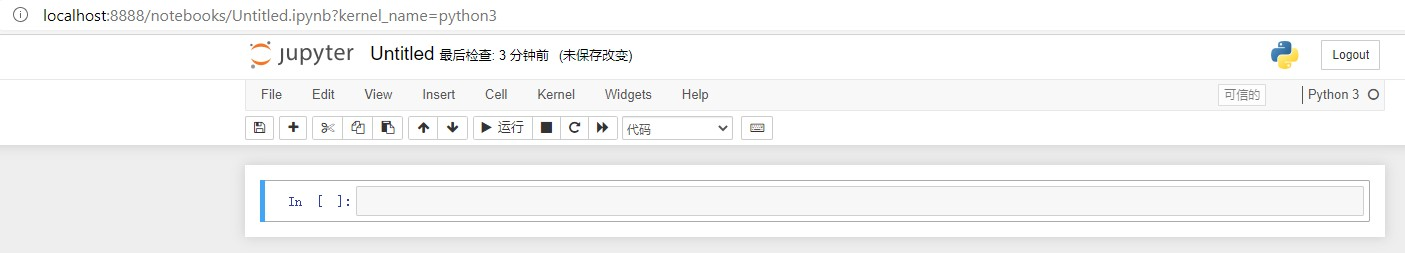(3) 输入如下代码并运行，结果如代码下面的图：

from IPython.display import display, Latex
import sympy as sy
sy.init_printing()

x,y=sy.symbols("x y")
f=x**2+3*x-5
display(Latex(f"$$f(x)={sy.latex(f)}$$"))

xx=3
sy.pprint(f"f({xx})={f.subs({x:xx})}")
yy=4
f1=sy.sqrt(x**2+y**2)

display(Latex(f"$$f_1(x,y)={sy.latex(f1)}$$"))
sy.pprint(f"f1({xx},{yy})={f1.subs({x:xx, y:yy})}")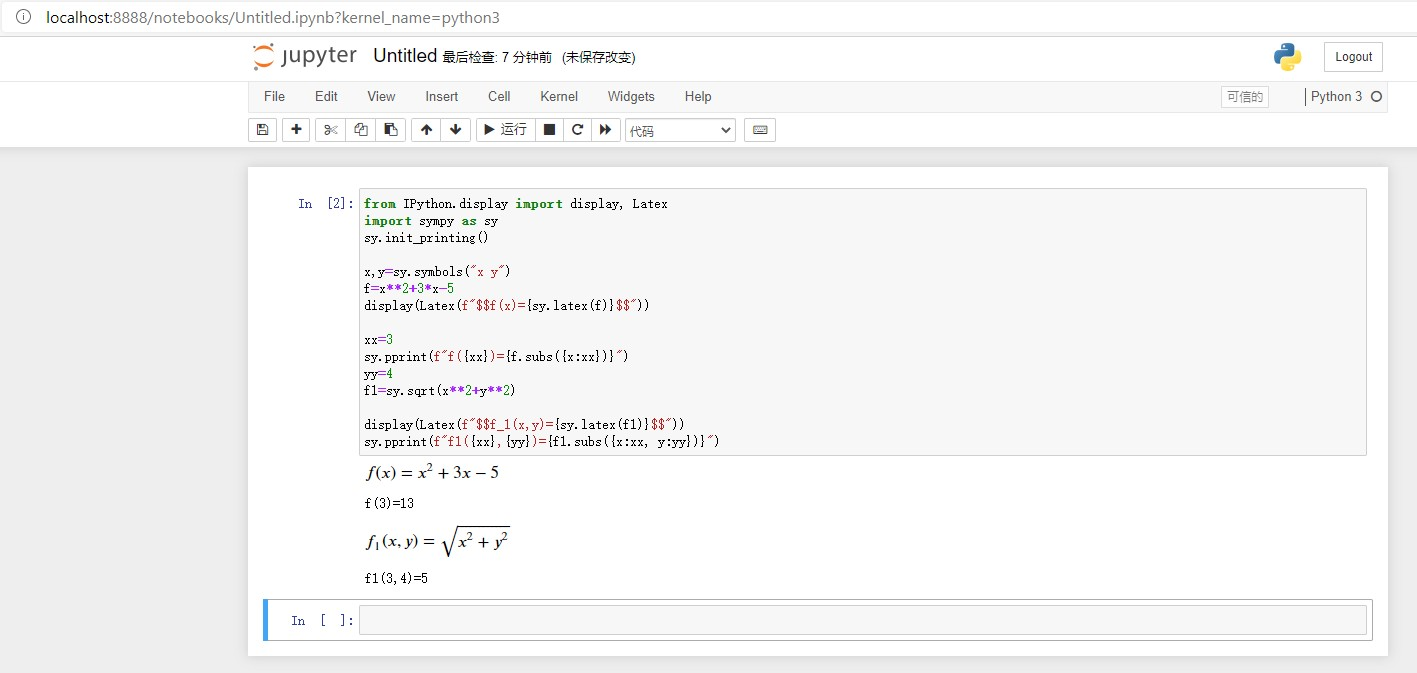三、更多功能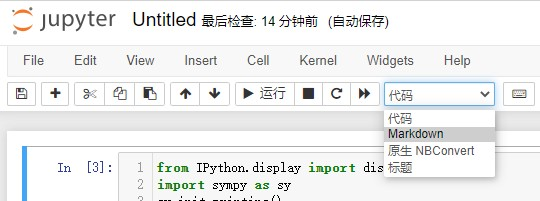如上图，jupyter 还支持更 MarkDown 等更多功能，使用方法也是动手弄一弄就会。结合这些功能一起，就能理解为什么叫 jupyter notebook 了，这实在是做代码笔记的不二之选。

简书上有一篇《Jupyter Notebook介绍、安装及使用教程》非常详细地讲述了 jupter 从安装使用以及加入其他功能插件的各种操作。

## PS

对于数学工具，Sugar 推荐相似功能先学习 Matlab 再学 Python，Matlab 环境下查看变量和断点调试更方便。

## 关注作者

欢迎扫码关注我的公众号MultiMCU EDU### 喜欢本文求点赞，有打赏我会更有动力。

展开全文• Symbolic Math Toolbox MAPLIE占据符号软件的主导地位符号运算工具包通过调用Maple软件实现符号计算的 在符号计算中参与运算的是符号变量而不是数值使用字符串进行分析数值运算中必须先对变量赋值然
• ## MATLAB 符号运算

千次阅读 2020-07-18 16:37:09
MATLAB的符号运算虽然是数值运算的补充，但是它仍然是科学计算研究中不可替代的重要内容。与数值运算相比，符号运算不需要预先对变量赋值，其运算结果以标准的符号形式表达。比如说计算sin(π)，数值运算的结果是1....

## 前言

有时候，你可能会遇到较复杂的方程(组)，希望用MATLAB来求解。MATLAB的符号运算正好可用于求解方程(组)。此外，它还有许多其他功能。例如，展开和简化、因式分解以及微积分运算等。MATLAB的符号运算虽然是数值运算的补充，但是它仍然是科学计算研究中不可替代的重要内容。与数值运算相比，符号运算不需要预先对变量赋值，其运算结果以标准的符号形式表达。比如说计算sin(π)，数值运算的结果是1.2246e-16，符号运算的结果是0。前者是近似的，后者是精确的。

## 正文

MATLAB符号运算功能非常强大，本文只介绍大部分常用的符号运算功能。注：本文代码的运行环境是MATLAB R2016b。

### 1. 创建符号数、符号变量和符号矩阵

这一步骤是符号运算的第一步，后面的步骤都是在此基础上进行的。

%创建符号数 (只能用sym函数)
s0 = 1 / sym(7)              %符号数，不适合大型符号数
s1 = sym('1/7')              %符号数
s2 = sym('3 + 4i')           %符号复数

%创建符号变量 (sym函数和syms函数都行)
%--sym函数
s3 = sym('x')             　　%符号变量

%--syms函数
syms a b c                　　%创建多个符号变量，值为本身
syms(sym('[d e; e d]'))      %用已存在的符号变量矩阵创建多个符号变量

%创建符号矩阵 (sym函数和syms函数都行)
s4 = sym('[2 5 6; 9 8 6]')    %符号数矩阵
s5 = sym('x', [2 3])          %符号变量矩阵，矩阵内的元素不会被创建为符号变量
A = [a b c; c b a]            %用已存在的符号变量创建符号变量矩阵
% syms A B [2 3]              %仅2017及以上版本支持，同时创建多个符号矩阵

代码运行结果如下。可以看到s5是一个2x3的符号变量矩阵，但矩阵内元素不会被创建成符号变量。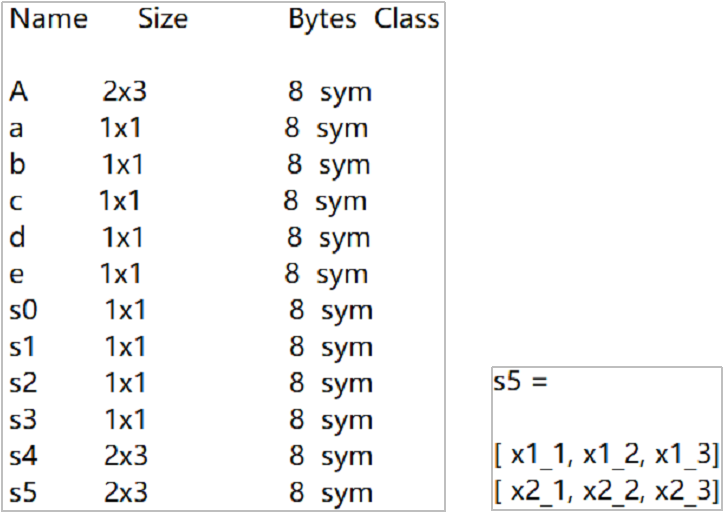### 2. 创建符号表达式、符号函数

要表达一个数学函数可以用符号函数或符号表达式。

%创建符号表达式
syms x y
f = x^2 + 5*x + 4         　　　　　　　　　　   %符号表达式
subs(f, x, [2 1])             　　　　　　　　　 %分别将f中的x换成2和1
f2 = sym('x^2 + 2*x + 1 = 0')   　　　       　 %创建方程
k = piecewise(x <= 0, x + y, x > 0, x - y);    %分段符号表达式
%更多分段符号表达式/函数的介绍详见：https://ww2.mathworks.cn/help/symbolic/piecewise.html#responsive_offcanvas

%创建符号函数
%--用syms函数
syms G(x, y) F(z)    %创建多个符号函数，变量名为括号前字母, 值为本身，其自变量也创建对应的符号变量
F(z) = z^2 + 5*z + 4                　　　　　　　　%符号函数
F([2 1])                                    　　　 %分别计算F(2)和F(1)
G(x, y) = piecewise(x <= 0, x + y, x > 0, x - y); %分段符号函数，有多个条件用&&连接

%--用symfun函数
syms x y
g = symfun(x^2 + y^2, [x y])   %因变量 = symfun(公式，[自变量1 自变量2])
g([2 3], [1 2])                %分别计算g(2, 1)和g(3, 2)

代码运行结果如下。从图中可以看出符号表达式与符号函数之间的区别，它们的类型不同，且计算函数值的方式的也不同。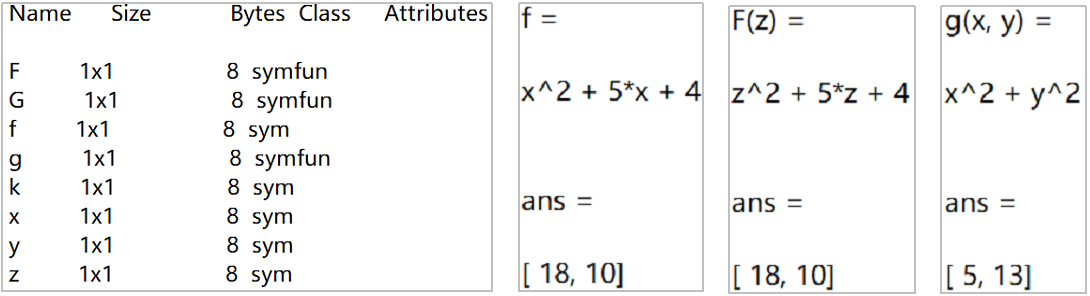### 3. 符号运算中的基本运算

符号运算是精确计算，不会产生截断误差。并且可以根据需要，给出完全的封闭解或任意精度的数值解。本节讨论符号运算中的基本运算，可分为算术运算和关系运算。

3.1. 算术运算

数值运算中的一些运算符，例如‘+’、‘-’、‘*’、‘/’、’.*‘、’./‘、‘^’等，在符号运算中也可以使用。由于比较简单，且和数值运算相同，这里不做展示。

3.2 关系运算

关系运算符在符号运算中也可以使用，包括’>‘、’>=‘、’<‘、’<=‘、’==‘、’~=‘等。但是，在符号运算中这些运算并不是用来做关系比较，而是通过这些关系运算符构建关系式，作为后续操作（例如，解方程）的限制条件。若要对两个符号式做关系比较，可以使用函数isAlways、isequal或isequaln。

%关系运算
syms x
assume(x > 2);                                      %假设x是大于2的
solve((x + 1)*(x - 1)*(x - 2)*(x - 4)*(x - 6) == 0) %在设定的条件下，解方程

%函数isAlways、isequal以及isequaln
%--isAlways：判断两个符号式构成的关系比较式的真假
A1 = [x, sym(9); 2*x^2, tan(x)];
B1 = [2*x, sym(6); x^2, sin(x)/cos(x)];
res1 = isAlways(A1  > B1)                    %对于数组情况，分别比较相应元素，结果为同等大小的logical数组

%--isequal和isequaln：都是判断输入的两个符号式是否相等。但前者将NAN视为不相等，后者将NAN视为相等
A2 = [x+1, nan]
B2 = [x+1, nan]
res2 = isequal(A2, B2)
res3 = isequaln(A2, B2)                　　　%对于数组情况，相应元素全部相等，返回1；反之，返回0

代码运行结果如下。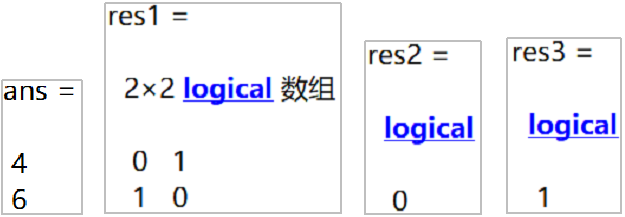### 4. 符号运算的主要功能

MATLAB符号运算提供了许多数学操作，例如解方程、微积分、线性代数运算、简化、变换等。本节将简单介绍微积分、解方程以及变换，更多详细功能介绍请看官方帮助文档

4.1 微积分

微积分是数学中非常重要的分析工具。MATALB提供了许多微积分的相关操作，这里简单介绍求极限、微分以及积分。详细功能见微积分帮助文档

%求极限
syms x
f1 = limit((exp(x) - 1) / x, x, 0)        %计算x逼近0时，f的双向极限值

%微分
syms x y
f2 = diff(sin(x*y), x, y)                 %f先对x求导，再对y求导

%积分
syms x y
f3 = x / (y^2 + 1);
res1 = int(f3, x)            %f关于x的不定积分
res2 = int(f3, x, 1, 3)      %f关于x在[1, 3]上的定积分
%changeIntegrationVariable函数：换元积分法
%integrateByParts函数：分部积分法

代码运行结果如下。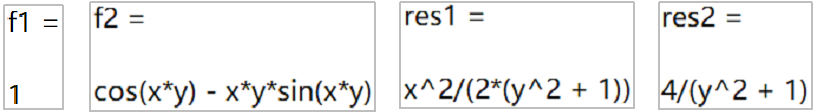4.2 解方程

利用MATALB可以求解多种方程（组），例如线性方程、非线性方程、微分方程等。详见方程求解帮助文档

%线性方程：所有未知数都是一次的。可用矩阵形式表示。（用linsolve）
syms x y z
eqns = [x+y-2*z == 0, x+y+z == 1, 2*y-z == -5];
[A, b] = equationsToMatrix(eqns)    　　　　　　　　 %将线性方程转为矩阵形式
X = linsolve(A, b)                             　　%linsolve函数大的对象是矩阵形式的线性方程

%非线性方程：（用solve函数或vpasolve函数）
%--求解析解
syms x y
eqns = [2*x^2 + y^2 == 0, 2*x - y == 4];
[solx soly] = solve(eqns, [x y])

%--求数值解
syms x
eqn = x^6 - x^2 == 2;
s1 = vpasolve(eqn, x)                %不指定求解范围
s2 = vpasolve(eqn, x, [-3, 3])       %指定求解范围

%常微分方程：未知函数是一元函数的微分方程称作常微分方程
syms y(t) a b
eqn = diff(y, t, 2) == 2 * a^2 * y;  %二阶常微分方程
diffY = diff(y, t);
cond = [y(0)==b, diffY(0)==1];       %初始条件
ySol(t) = dsolve(eqn, cond)

代码运行结果如下。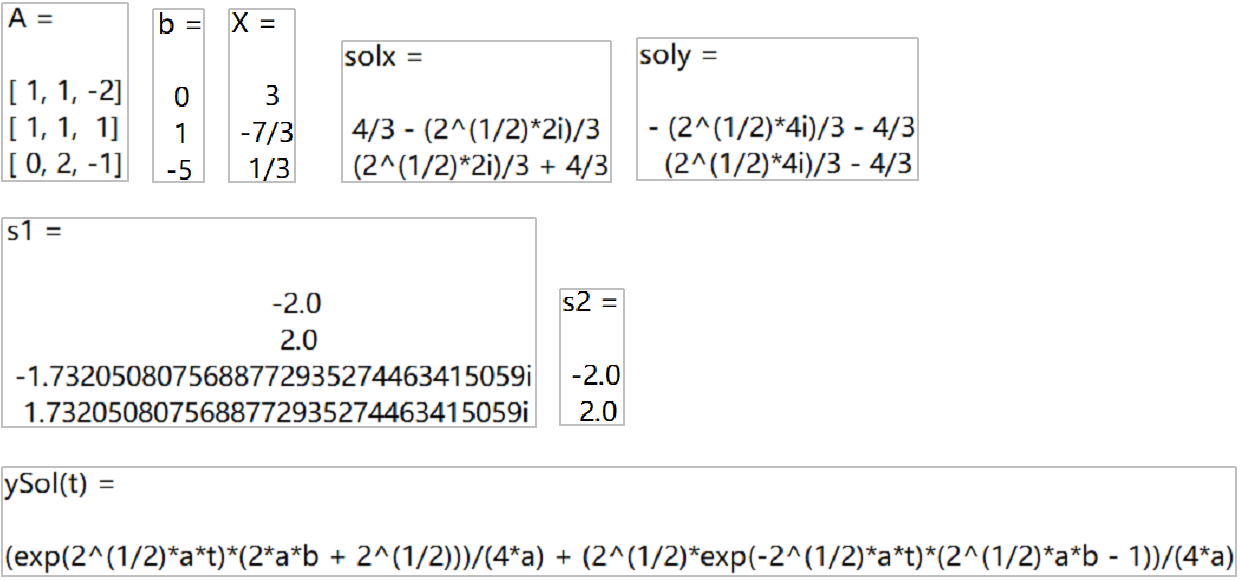4.3 变换

傅里叶变换、拉普拉斯变换以及Z变换是数学中的常用变换，也是对于工科学生很重要的变换。MATLBA为上述变换都提供了相应的函数来实现。下面开始介绍这些函数的简单使用，每个变换的定义不进行说明，详细说明见变换函数的帮助文档

%傅里叶变换
syms t
f = cos(2 * t);
f_FT = fourier(f)         %傅里叶变换
f_IFT = ifourier(f_FT)    %傅里叶逆变换
% fourier(f, y)           %指定变换变量，默认是w
% fourier(f, t, y)        %指定自变量和变换变量，默认自变量可以用symvar查看

%拉普拉斯变换
syms t
f = 2 * t;
f_LT = laplace(f)         %拉普拉斯变换
f_ILT = ilaplace(f_LFT)   %拉普拉斯逆变换
% laplace(f, y)           %指定变换变量，默认是s
% laplace(f, t, y)        %指定自变量和变换变量

%Z变换
syms n z
f = sin(n);
f_ZT = ztrans(f)          %Z变换
f_IZT = iztrans(f_ZT)     %逆Z变换
% ztrans(f, y)            %指定变换变量，默认是z
% ztrans(f, t, y)         %指定自变量和变换变量

代码运行结果如下。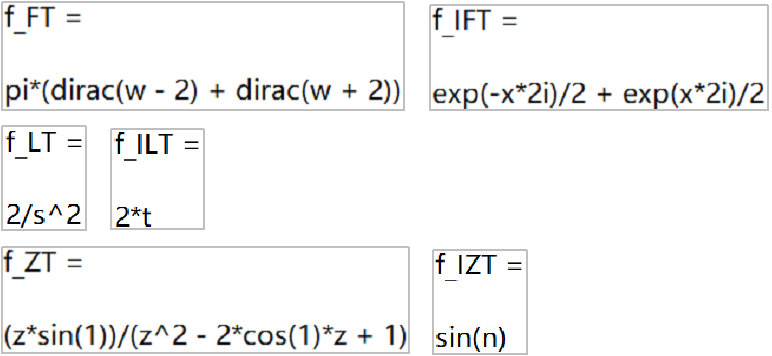### 5. 绘图

对于前面提到的符号表达式、符号方程、符号函数等，MATLBA提供了丰富的绘图函数来绘制它们。这些图可以是2D或3D形式的直线、轮廓线、曲面或网格。甚至，还可以创建动画图。下面展示2D和3D形式的曲线图，更详细说明见绘图帮助文档

syms x
fplot(tan(x) * sin(x));    　　%2D曲线

syms t
xt = exp(-t/10).*sin(5*t);    %参数方程
yt = exp(-t/10).*cos(5*t);
zt = t;
figure
fplot3(xt, yt, zt)            %3D曲线

代码运行结果如下。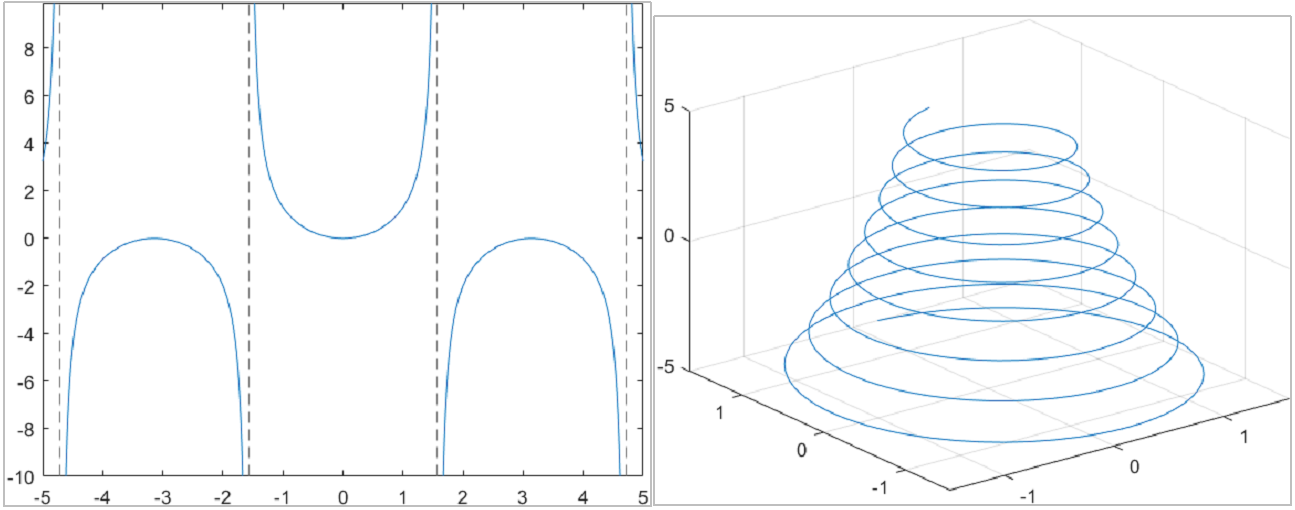博主：虔诚~似锦（主博客）

个性签名：如果你愿意努力，人生最坏的结果也不过是大器晚成。

------------------------------------------------------------------------------------

如果这篇文章对你帮助的话，记得在下方点赞哦，博主在此感谢！🎉🎉🎉

如果对这篇文章有疑问，请在评论区指出，欢迎探讨，共同进步。😄😄😄

展开全文• Matlab符号运算讲义-Matlab 符号运算.ppt Matlab 符号运算讲义 应该可以下载，没法下载的吱一声我给发邮箱去！ 分享才能使人快乐！matlab
• 第3章MATLAB符号计算符号计算则是可以对未赋值的符号对象(可以是常数、变量、表达式)进行运算和处理。MATLAB具有符号数学工具箱(Symbolic Math Toolbox)，将符号运算结合到MATLAB的数值运算环境。符号数学工具箱是...
• ## 利用MATLAB进行符号运算。

千次阅读 多人点赞 2020-08-18 21:01:11
本文是《科学计算与MATLAB语言》专题七第1小节的学习笔记，如果大家有时间的话，可以去听听课，...从符号对象的建立、运算符号矩阵的运算，本文都有详细的记录，每个代码我都跑过一遍，可以直接复制到MATLAB中运行。matlab
• 请注意在C和C++中，在整数的双目运算符中，如果两个整数数据的符号类型不一致，那么在运算时会转换成无符号进行运算。即原先有符号的会转换成无符号的进行运算。 举例： uint32_t a = 10; int32_t b = -1; if...
• matlab符号运算函数大全，讲述常用的matlab符号运算函数，包括solve等一系列运算函数
• MATLAB符号运算及其应用.pdf MATLAB符号运算及其应用.pdf MATLAB符号运算及其应用.pdf MATLAB符号运算及其应用.pdf MATLAB符号运算及其应用.pdf MATLAB符号运算及其应用.pdfmatlab
• 七、MATLAB符号计算 7.1 符号对象 符号对象的计算结果是一个更加精确的表达式。数值计算的结果往往是值。 7.1.1 符号对象的建立 符号对象名=sym(A)，A可以是数值常量、矩阵或数值表达式**（不加单引号），符号对象可...
• ## 学习matlab（七）——符号计算

万次阅读 多人点赞 2020-02-16 23:48:01
在MATLAB中，提供了强大的符号运算功能，并且有...在MATLAB中，符号计算的函数主要分为以下几类:符号表达式、符号矩阵操作、符号微积分、符号积分变换、符号方程求解、符号函数的绘图、图形化符号函数计算器 符号...
• 实用标准文案 实验五 Matlab 多项式和符号运算 一实验目的 1掌握 Matlab 多项式的运算 2了解符号运算 二实验内容 1将多项式 P(x) ( x 2( x 3( x 7( x 1) 化为 x 的降幂排列 syms x; y=(x-2(x+3(x-7(x+1; expand(y) ...
• 实验四 MATLAB 数值计算与符号计算 一实验目的 1.掌握数据插值和曲线拟合的方法 2.掌握求数值导数和数值积分的方法 3.掌握代数方程数值求解的方法 4.掌握常微分方程数值求解的方法 5.掌握求解优化问题的方法 6.掌握...
•matlab符号运算
• ## MATLAB中符号运算和数值运算的区别

千次阅读 多人点赞 2020-10-10 22:41:29
两者的根本区别是 : 数值计算的表达式、矩阵变量中不允许有未定义的自由变量 , 而符号计算可以含有未定义的符号变量。对于一般的程序设计软件如 C, C + + 等语言实现数值计算还可以 , 但是实现符号计算并不是一件...
• %m,n为符号式，即用符号代替一些式子 int(int(f,a),x);%对a求一次积分后，作为一个新的函数，再对x求一次积分 例： 已知f(x)=ax^2+bx+c，求f(x)的积分 syms a x b c; %变量分别为a x b c f=a*x^2+b*x+c; int(f); %f...matlab
• MATLAB的符号计算 matlab的符号计算是通过sym、syms 函数去创建符号对象或者符号表达式。例如一元二次函数我们便可以通过syms 函数创建。 syms a b c x y z f1 = a * x^2 + b * x + c; f2 = sin(x) * cos(y); f3 ...matlab
• MATLAB程序设计教程(9)——MATLAB符号计算第9章MATLAB符号计算9.1 符号对象9.2 符号微积分9.3 级 数9.4 符号方程求解9.1符号对象9.1.1 建立符号对象1．建立符号变量和符号常量MATLAB提供了两个建立符号对象的函数：...
• MATLAB变量和数据操作 MATLAB矩阵及其操作 MATLAB矩阵运算...